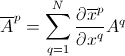## Pages

### 6. Co-ordinate Transformation

Let $(x^{1}, x^{2}, x^{3}...)$ and $(\overline{x}^{1}, \overline{x}^{2}, \overline{x}^{3}...)$ be the coordinates of a point in the two different frames of reference. Let us also consider that the coordinate can be interchanged from one system to the other.
i.e. $x^{n}= x^{n}(\overline{x}^{1}, \overline{x}^{2}, \overline{x}^{3}...)$, where $n$ is any natural number. Similarly the reverse transformation follows too.
As we have seen in the previous notes that there can be two different representation of the vectors in any coordinate system. They are the covariant vectors and the contravariant vectors. The short notes on this can be found here. It must be noted that only one of the basis from either of the two is used for solving problems.
So, considering the Contravariant Vectors, how do these transform?
Suppose $N$ quantities $(A^{1}, A^{2}, A^{3}.....,A^{N})$ in a coordinate system $(x^{1}, x^{2}, x^{3}....x^{N})$ are related to $N$ other quantities $(\overline{A}^{1}, \overline{A}^{2}, \overline{A}^{3}.....\overline{A}^{N})$ in another coordinate system $(\overline{x}^{1}, \overline{x}^{2}, \overline{x}^{3}...)$ by the transformation equation,Then they are called components of contravariant vector or contravariant tensor of the first rank or first order.
Similarly for the Covariant vectors,
This is the transformation of the components of the covariant vector or covariant tensor of the first rank or first order.
Hence, these are the two basic coordinate transformation between the different coordinate sytem.